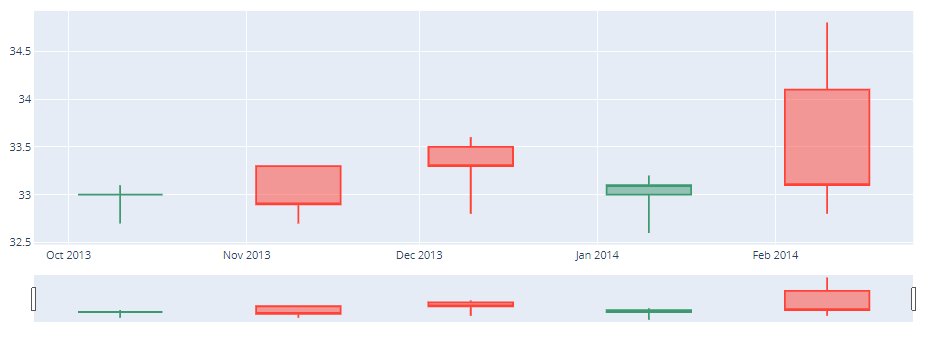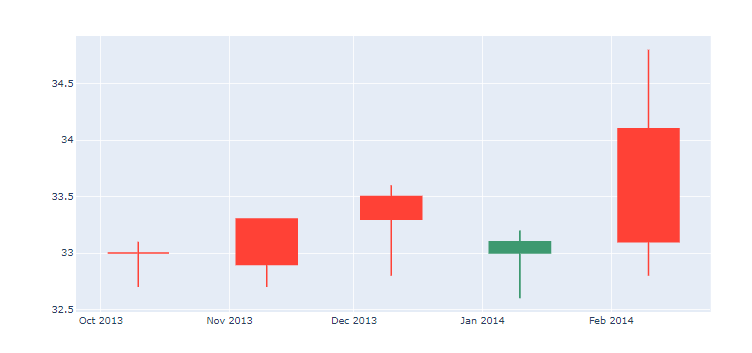GeeksforGeeks App
Open AppBrowser
Continue

# plotly.figure_factory.create_candlestick() function in Python

Plotly library of Python can be very useful for data visualization and understanding the data simply and easily.

## plotly.figure_factory.create_candlestick

The candlestick chart is a style of financial chart that describes o for a given x coordinate (most likely time). The boxes represent the spread between the open and close values and the lines represent the spread between the low and high values.

Syntax: plotly.figure_factory.create_candlestick(open, high, low, close, dates=None, direction=’both’, **kwargs)

Parameters

open: it is used for opening values

high: it is used for high values

low: it is used for low values

close: it is used for closing values

dates: it is used for list of datetime objects. Default: None

direction: it can be used for both increasing and decreasing. When the direction is ‘increasing’, the returned figure consists of all candlesticks where the close value is greater than the corresponding open value, and when the direction is ‘decreasing’, the returned figure consists of all candlesticks where the close value is less than or equal to the corresponding open value. When the direction is ‘both’, both increasing and decreasing candlesticks are returned. Default: ‘both’

kwargs – it describe other attributes about the ohlc Scatter trace such as the color or the legend name. For more information on valid kwargs call help(plotly.graph_objects.Scatter)

Example 1: With datetime Objects

## Python3

 `import` `plotly.graph_objects as go``from` `datetime ``import` `datetime`` ` `open_data ``=` `[``33.0``, ``33.3``, ``33.5``, ``33.0``, ``34.1``]``high_data ``=` `[``33.1``, ``33.3``, ``33.6``, ``33.2``, ``34.8``]``low_data ``=` `[``32.7``, ``32.7``, ``32.8``, ``32.6``, ``32.8``]``close_data ``=` `[``33.0``, ``32.9``, ``33.3``, ``33.1``, ``33.1``]``dates ``=` `[datetime(year``=``2013``, month``=``10``, day``=``10``),``         ``datetime(year``=``2013``, month``=``11``, day``=``10``),``         ``datetime(year``=``2013``, month``=``12``, day``=``10``),``         ``datetime(year``=``2014``, month``=``1``, day``=``10``),``         ``datetime(year``=``2014``, month``=``2``, day``=``10``)]`` ` `fig ``=` `go.Figure(data``=``[go.Candlestick(x``=``dates,``                       ``open``=``open_data, high``=``high_data,``                       ``low``=``low_data, close``=``close_data)])`` ` `fig.show()`

Output:Example 2: Candlestick chart with datetime objects

## Python3

 `from` `plotly.figure_factory ``import` `create_candlestick``from` `datetime ``import` `datetime``# Add data``open_data ``=` `[``33.0``, ``33.3``, ``33.5``, ``33.0``, ``34.1``]``high_data ``=` `[``33.1``, ``33.3``, ``33.6``, ``33.2``, ``34.8``]``low_data ``=` `[``32.7``, ``32.7``, ``32.8``, ``32.6``, ``32.8``]``close_data ``=` `[``33.0``, ``32.9``, ``33.3``, ``33.1``, ``33.1``]``dates ``=` `[datetime(year``=``2013``, month``=``10``, day``=``10``),``         ``datetime(year``=``2013``, month``=``11``, day``=``10``),``         ``datetime(year``=``2013``, month``=``12``, day``=``10``),``         ``datetime(year``=``2014``, month``=``1``, day``=``10``),``         ``datetime(year``=``2014``, month``=``2``, day``=``10``)]``# Create ohlc``fig ``=` `create_candlestick(open_data, high_data,``    ``low_data, close_data, dates``=``dates)``fig.show()`

Output:My Personal Notes arrow_drop_up